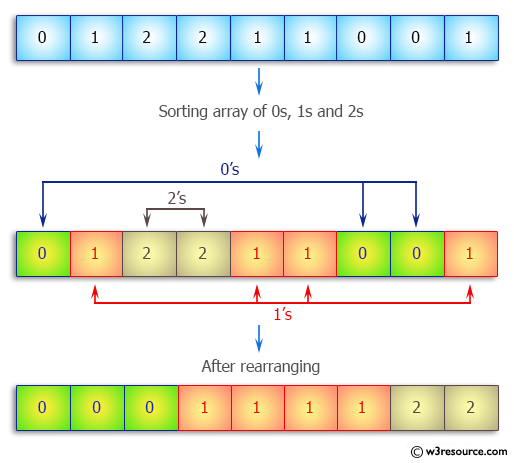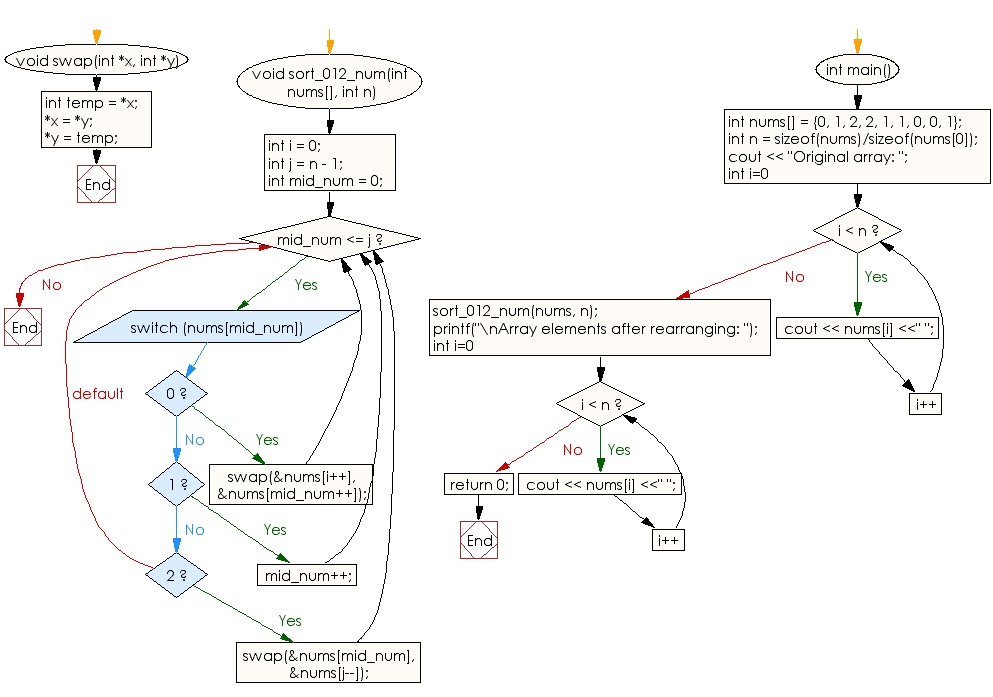﻿ C++ Exercises: Sort a given array of 0s, 1s and 2s - w3resource# C++ Exercises: Sort a given array of 0s, 1s and 2s

## C++ Array: Exercise-16 with Solution

Write a C++ program to sort a given array of 0s, 1s and 2s. In the final array put all 0s first, then all 1s and all 2s in last.

Pictorial Presentation:Sample Solution:

C++ Code :

``````#include <iostream>
using namespace std;

void swap(int *x, int *y)
{
int temp = *x;
*x = *y;
*y = temp;
}

void sort_012_num(int nums[], int n)
{
int i = 0;
int j = n - 1;
int mid_num = 0;

while (mid_num <= j)
{
switch (nums[mid_num])
{
case 0:
swap(&nums[i++], &nums[mid_num++]);
break;
case 1:
mid_num++;
break;
case 2:
swap(&nums[mid_num], &nums[j--]);
break;
}
}
}

int main()
{
int nums[] = {0, 1, 2, 2, 1, 1, 0, 0, 1};
int n = sizeof(nums)/sizeof(nums);
cout << "Original array: ";
for (int i=0; i < n; i++)
cout << nums[i] <<" ";
sort_012_num(nums, n);

printf("\nArray elements after rearranging: ");
for (int i=0; i < n; i++)
cout << nums[i] <<" ";
return 0;
}
``````

Sample Output:

```Original array: 0 1 2 2 1 1 0 0 1
Array elements after rearranging: 0 0 0 1 1 1 1 2 2
```

Flowchart:C++ Code Editor:

What is the difficulty level of this exercise?

Test your Programming skills with w3resource's quiz.

﻿